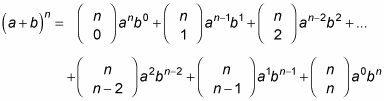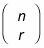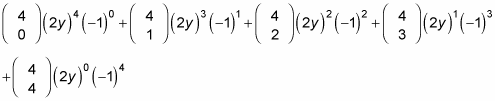##### Differential Equations For DummiesA binomial is a polynomial with exactly two terms. Multiplying out a binomial raised to a power is called binomial expansion. Your pre-calculus teacher may ask you to use the binomial theorem to find the coefficients of this expansion.

Expanding many binomials takes a rather extensive application of the distributive property and quite a bit of time. Multiplying two binomials is easy if you use the FOIL method, and multiplying three binomials doesn't take much more effort. Multiplying ten binomials, however, takes long enough that you may end up quitting short of the halfway point. And if you make a mistake somewhere along the line, it snowballs and affects every subsequent step.

Therefore, in the interest of saving bushels of time and energy, here is the binomial theorem. If you need to find the entire expansion for a binomial, this theorem is the greatest thing since sliced bread:This formula gives you a very abstract view of how to multiply a binomial n times. It's quite hard to read, actually. But this form is the way your textbook shows it to you.

Fortunately, the actual use of this formula is not as hard as it looks. Eachcomes from a combination formula and gives you the coefficients for each term (they're sometimes called binomial coefficients).

For example, to find (2y – 1)4, you start off the binomial theorem by replacing a with 2y, b with –1, and n with 4 to get:The binomial theorem looks extremely intimidating, but it becomes much simpler if you break it down into smaller steps and examine the parts. There are a few things to be aware of so that you don't get confused along the way; after you have all this info straightened out, your task will seem much more manageable:

• The binomial coefficientswon't necessarily be the coefficients in your final answer. You're raising each monomial to a power, including any coefficients attached to each of them.

• The theorem is written as the sum of two monomials, so if your task is to expand the difference of two monomials, the terms in your final answer should alternate between positive and negative numbers.

• The exponent of the first monomial begins at n and decreases by 1 with each sequential term until it reaches 0 at the last term. The exponent of the second monomial begins at 0 and increases by 1 each time until it reaches n at the last term.

• The exponents of both monomials add to n — unless the monomials themselves are also raised to powers.